﻿ 基于扩张观测器的船舶动力定位系统反演滑模变结构控制
 舰船科学技术2017, Vol. 39Issue (2): 103-107PDF

Back-stepping sliding mode control of ship dynamic positioning system based on extended state observer
JIN Yue, YU Meng-hong, YUAN Wei, FAN Ji-sheng
School of Electronic and Information, Jiangsu University of Science and Technology, Zhenjiang 212003, China
Abstract: Aiming at the ship dynamic positioning control system in the offshore platform, In view of the advantages of back-stepping sliding mode control and extended observer, a sliding mode control method for dynamic positioning of ship based on extended observer is proposed. Considering the system of unknown external disturbances and parameters of ship model uncertainty, the system is divided as the observer of the inner ring and the outer ring controller. First, using the extended state observer to estimate the unknown state and uncertainty of the system which are compensated in the outer ring of the back-stepping sliding mode control then, finally Lyapunov method is used to demonstrate the system's stability. The ship point control simulation experiment shows that back-stepping sliding mode controller based on extended state observer with strong robustness and control performance can make the ship's surge position, sway position and swing angle gradually keep in the expected value. It can effectively suppress the chattering problem in the conventional sliding mode control and is beneficial to the application of ship engineering.
Key words: dynamic positioning control     extended state observer     back-stepping sliding mode control
0 引言

1 船舶数学模型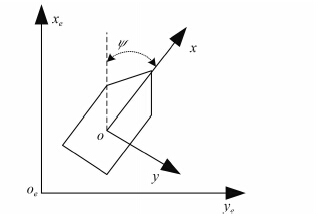图 1 大地坐标系和船体坐标系 Fig. 1 Geodetic coordinate system and ship coordinate system

 $\left\{ \begin{array}{l} \dot \eta = R\left( \psi \right)v \text{，}\\ M\dot \nu+D\nu = {R^{\rm T}}(\psi )b+\tau \text{。} \end{array} \right.$ (1)

 $\begin{array}{l} { R}(\psi ) = \left[ {\begin{array}{*{20}{l}} {\cos \psi } & { - \sin \psi } & 0\\ {\sin \psi } & {\cos \psi } & 0\\ 0 & 0 & 1 \end{array}} \right]\text{，}\\ { M} = \left[ {\begin{array}{*{20}{c}} {m - {X_{\dot u}}} & 0 & 0\\ 0 & {m - {Y_{\dot v}}} & {m{x_g} - {Y_{\dot r}}}\\ 0 & {m{x_g} - {N_{\dot v}}} & {{I_z} - {N_{\dot r}}} \end{array}} \right]\text{，}\\ { D} = \left[ {\begin{array}{*{20}{c}} { - {X_u}} & 0 & 0\\ 0 & { - {Y_v}} & { - {Y_r}}\\ 0 & { - {N_v}} & { - {N_r}} \end{array}} \right]\text{。} \end{array}$

2 基于扩张观测器的反演滑模变结构控制系统的设计

2.1 扩张观测器的设计

 $\left\{ \begin{array}{l} {x^{\left( n \right)}} = f\left( {x,{x^{\left( 1 \right)}}, \cdots ,{x^{\left( {n - 1} \right)}},t} \right)+w\left( t \right)+bu \text{，}\\ y = x\left( t \right)\text{。} \end{array} \right.$ (2)

$a\left( t \right) = f\left( {x,{x^{\left( 1 \right)}}, \cdots ,{x^{\left( {n - 1} \right)}},t} \right)+w\left( t \right)$，则n+1 阶 ESO 的一般形式为：

 $\left\{ \begin{array}{l} {{\dot z}_1} = {z_2} - {g_1}\left( {{z_1} - x\left( t \right)} \right)\text{，}\\ \vdots \\ {{\dot z}_n} = {z_{n+1}} - {g_n}\left( {{z_1} - x\left( t \right)} \right)+bu \text{，}\\ {{\dot z}_{n+1}} = - {g_{n+1}}\left( {{z_1} - x\left( t \right)} \right)\text{。} \end{array} \right.$ (3)

 $\begin{array}{l} fal\left( {\varepsilon ,\alpha ,\delta } \right) = \left\{ \begin{array}{l} {\left| \varepsilon \right|^\alpha }sat\left( \varepsilon \right),\left| \varepsilon \right| > \delta \text{，}\\ sign\left( \varepsilon \right),\varepsilon \leqslant \delta \text{，} \end{array} \right.\\ sat\left( \varepsilon \right) = \left\{ \begin{array}{l} \varepsilon /\varsigma ,\left| \varepsilon \right| \leqslant \varsigma \text{，}\\ sign\left( \varepsilon \right),\left| \varepsilon \right| > \varsigma \text{。} \end{array} \right. \end{array}$ (4)

 $\left\{ \begin{array}{l} {{\dot z}_1} = {z_2} - {\beta _1}{\varepsilon _1}\text{，}\\ {{\dot z}_2} = {z_3} - {\beta _2}fal\left( {{\varepsilon _1},{\alpha _1},\delta } \right)+bu\text{，}\\ {{\dot z}_2} = - {\beta _3}fal\left( {{\varepsilon _1},{\alpha _2},\delta } \right)\text{。} \end{array} \right.$ (5)

2.2 反演滑模控制器的设计

 ${ M}{{ R}^{ - 1}}\left( \varphi \right)\ddot \eta+{ M}{\dot { R}^{ - 1}}\left( \varphi \right)\dot \eta+{ D}{{ R}^{ - 1}}\left( \varphi \right)\dot \eta = \tau+d\left( t \right)\text{，}$ (6)

 $\ddot \eta = { A}\dot \eta+{ B}\left( {\tau+d\left( t \right)} \right)\text{，}$ (7)

${v^*} \!=\! { R}\left( \varphi \right)v \Rightarrow \dot \eta \!=\! {v^*}$，对式（1）进行简单调整：

 $\left\{ \begin{array}{l} \dot \eta = {v^*}\text{，}\\ {{\dot v}^*} = { A}{v^*}+{ B}\left( {\tau+d\left( t \right)} \right)\text{。} \end{array} \right.$ (8)

1） 船舶轨迹跟踪误差为z1 =η-ηdηd 为船舶目标位置，则 ${\dot z_1} = {v^*} - {\dot \eta _d}$

 ${V_1} = \frac{1}{2}z_1^2\text{，}$ (9)

 ${\dot V_1} = {z_1}{\dot z_1} = {z_1}{z_2} - {c_1}z_1^2\text{，}$ (10)

 $\sigma = {k_1}{z_1}+{z_2}\text{。}$ (11)

 $\sigma \!=\! {k_1}{z_1} \!+\! {z_2} \!=\! {k_1}{z_1} \!+\! {\dot z_1}+{c_1}{z_1} = \left( {{k_1}+{c_1}} \right){z_1}+{\dot z_1}\text{。}$ (12)

2） 定义 Lyapunov 函数：

 ${V_2} = {V_1}+\frac{1}{2}{\sigma ^2}\text{，}$ (13)

 $\begin{split}\\[-12pt] {{\dot V}_2} = & {{\dot V}_1}+\sigma \dot \sigma = {z_1}{z_2} - {c_1}z_1^2+\sigma \dot \sigma = \\ & {z_1}{z_2} - {c_1}z_1^2+\sigma \left( {{k_1}{{\dot z}_1}+{{\dot z}_2}} \right) = \\ & {z_1}{z_2} \!-\! {c_1}z_1^2 \!+\! \sigma \left( {{k_1}\left( {{z_2} \!-\! {c_1}{z_1}} \right) \!+\! {v^*} \!-\! {{\ddot \eta }_d} \!+\! {c_1}{{\dot z}_1}} \right) = \\ & {z_1}{z_2}\! - \!{c_1}z_1^2 \!+\! \sigma \left( {{k_1}\left( {{z_2} \!-\! {c_1}{z_1}} \right) \!+\! A\left( {{z_2} \!+\! {{\dot \eta }_d} \!-\! {c_1}{z_1}} \right)} +\right.\\ & \left. { Bu+F - {{\ddot \eta }_d}+{c_1}{{\dot z}_1}} \right)\text{。} \end{split}$ (14)

 $\begin{split}\\[-12pt] u = & {{ B}^{ - 1}}\left( { - {k_1}\left( {{z_2} - {c_1}{z_1}} \right) - A\left( {{z_2}+{{\dot \eta }_d} - {c_1}{z_1}} \right) - } \right.\\ & \left. {\bar F{\mathop{\rm sgn}} \left( \sigma \right)+{{\ddot \eta }_d} - {c_1}{{\dot z}_1} - h\left( {\sigma+\beta {\mathop{\rm sgn}} \left( \sigma \right)} \right)} \right)\text{。} \end{split}$ (15)

3 系统稳定性分析

 $\begin{split}\\[-12pt] {{\dot V}_2} = & {z_1}{z_2} - {c_1}z_1^2 - h{\sigma ^2} - h\beta \left| \sigma \right|+F\sigma - \\ & \bar F\left| \sigma \right| \leqslant - {c_1}z_1^2+{z_1}{z_2} - h{\sigma ^2} - h\beta \left| \sigma \right|\text{，} \end{split}$ (16)

 ${ Q} = \left[ {\begin{array}{*{20}{c}} {{c_1}+hk_1^2} & {h{k_1} - \frac{1}{2}}\\ {h{k_1} - \frac{1}{2}} & h \end{array}} \right]\text{，}$ (17)

 $\begin{split}\\[-12pt] {z^{\rm T}}{ Q}z = & \left[ \!\!\!{\begin{array}{*{20}{c}} {{z_1}} & {{z_2}} \end{array}}\!\! \right]\left[ \!\!{\begin{array}{*{20}{c}} {{c_1}+hk_1^2} & {h{k_1} - \frac{1}{2}}\\ {h{k_1} - \frac{1}{2}} & h \end{array}} \!\! \right]{\left[ \!\!{\begin{array}{*{20}{c}} {{z_1}} & {{z_2}} \end{array}}\!\! \right]^{\bf T}}= \\ & {\rm{ }} {c_1}z_1^2 - {z_1}{z_2}+hk_1^2z_1^2+2h{k_1}{z_1}{z_2}+hz_2^2 =\\ & {\rm{ }} {c_1}z_1^2 - {z_1}{z_2}+h{\sigma ^2}\text{，} \end{split}$ (18)

 ${\dot V_2} \leqslant - {z^{\bf T}}{ Q}z - h\beta \left| \sigma \right| \leqslant 0 \text{，}$ (19)

 $\left| { Q} \right| = h\left( {{c_1}+hk_1^2} \right) - {\left( {h{k_1} - \frac{1}{2}} \right)^2} = h\left( {{c_1}+{k_1}} \right) - \frac{1}{4}\text{。}$ (20)

4 仿真研究及结果表 1 仿真试验的主要参数 Tab.1 The main parameters of the simulation experiment

 $\begin{array}{l} { M} = \left[ {\begin{array}{*{20}{c}} {0.9270} & 0 & 0\\ 0 & {1.7502} & { - 0.1754}\\ 0 & { - 0.1754} & {0.1578} \end{array}} \right] \text{，}\\ { D} = \left[ {\begin{array}{*{20}{c}} {0.0558} & 0 & 0\\ 0 & {0.1183} & { - 0.0402}\\ 0 & { - 0.0151} & {0.0506} \end{array}} \right]\text{。} \end{array}$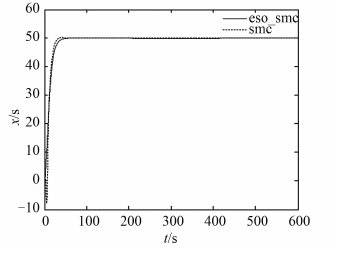图 2 船舶纵荡位置输出 Fig. 2 Output of the ship longitudinal swing position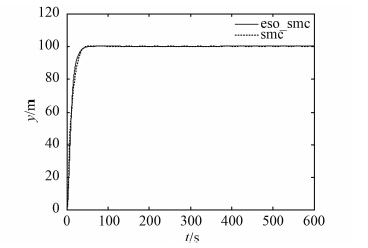图 3 船舶横荡位置输出 Fig. 3 Output of ship swing position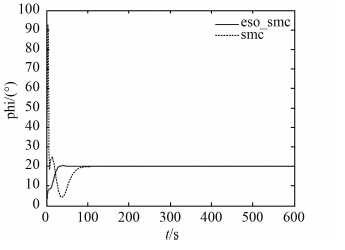图 4 船舶艏摇角度输出 Fig. 4 Output of ship yaw angle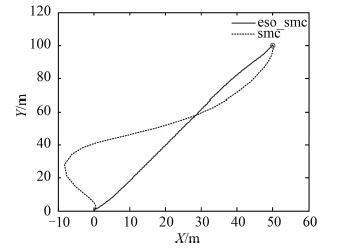图 5 船舶运动轨迹 Fig. 5 The track of the ship motion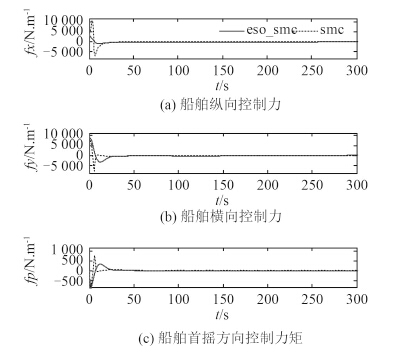图 6 船舶控制力、力矩 Fig. 6 Ship control force and control torque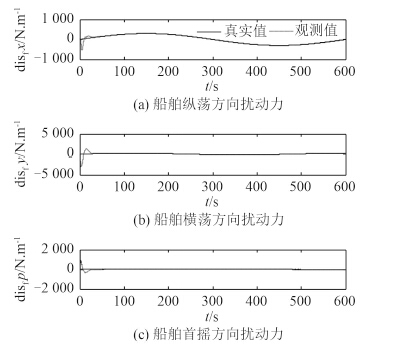图 7 船舶外界扰动力观测值 Fig. 7 Observation values of external disturbance
5 结语

  徐瑞萍, 高存臣. 基于线性反馈控制的一类混沌系统的同步[J]. 中国海洋大学学报(自然科学版), 2014 (5): 17. XU Rui-ping, GAO Cun-chen. Synchronization of a class of chaotic systems based on linear feedback control[J]. Journal of Ocean University of China (natural science edition), 2014 (5): 17.  李洪兴, 苗志宏, 王加银. 非线性系统的变论域稳定自适应模糊控制[J]. 中国科学(E辑), 2002, 32 (2): 211–223. LI Hong-xing, MIAO Zhi-hong, WANG Jia-yin. Stability and adaptive fuzzy control of nonlinear systems with variable universe adaptive fuzzy control[J]. China Science (E), 2002, 32 (2): 211–223.  刘金琨, 孙富春. 滑模变结构控制理论及其算法研究与进展[J]. 控制理论与应用, 2007, 24 (3): 407–418. LIU Jin-kun, SUN Fu-chun. Research and application of sliding mode variable structure control theory and its algorithm research and development[J]. Control Theory and Application, 2007, 24 (3): 407–418.  达飞鹏, 宋文忠. 基于模糊神经网络滑模控制器的一类非线性系统自适应控制[J]. 中国电机工程学报, 2002, 22 (5): 78–83. DA Fei-peng, SONG Wen-zhong. Based on the fuzzy neural network sliding mode controller for a class of nonlinear system adaptive control[J]. Proceedings of the CSEE, 2002, 22 (5): 78–83.  高峰. 船舶动力定位自抗扰控制及仿真的研究[D]. 大连:大连海事大学, 2013.  贾欣乐, 杨盐生. 船舶运动数学模型[M]. 大连: 大连海事出版社, 1999.  边信黔, 付明玉, 王元慧. 船舶动力定位[M]. 北京: 科学出版社, 2011.  CHEN Q, YU L, NAN Y R. 2013 J. Syst. Sci. Complex. 26940.  刘金棍. 滑模变结构控制Matlab仿真[M]. 北京: 清华大学出版社, 2005.